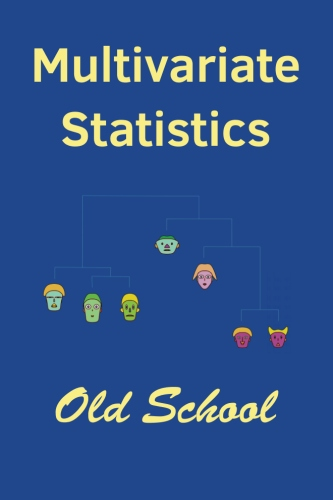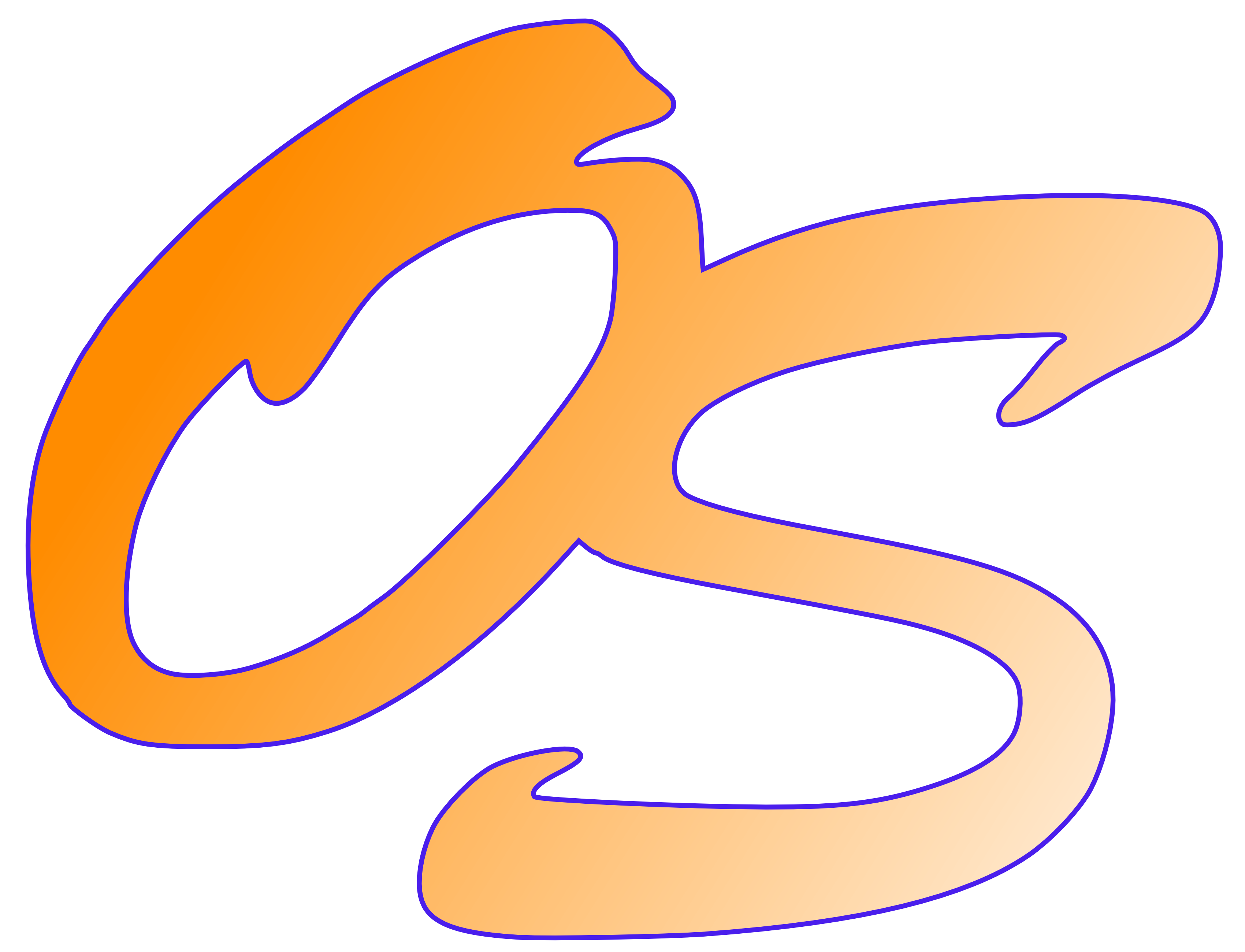# Multivariate Statistics: Old SchoolMultivariate Statistics: Old School is a mathematical and methodological introduction to multivariate statistical analysis. It presents the basic mathematical grounding that graduate statistics students need for future research, and important multivariate techniques useful to statisticians in general. This text was developed over many years by the author, John Marden, while teaching in the Department of Statistics, University of Illinois at Urbana-Champaign.

Topics include

• The multivariate normal and Wishart distributions
• Linear models, including multivariate regression and analysis of variance, and both-sides models (GMANOVA, repeated measures, growth curves)
• Linear algebra useful for multivariate statistics
• Covariance structures, including principal components, factor analysis, independence and conditional independence, and symmetry models
• Classification (linear and quadratic discrimination, trees, logistic regression)
• Clustering (K-means, model-based, hierarchical)
• Other techniques, including biplots, canonical correlations, and multidimensional scalingThe pdf version of the book. (Free!)

Buy a hard copy: Only \$29.95!

The associated R package: Balamuta & Marden

Some pdfs of slides used in class:

3D Spin

The Data Program has facilities for displaying interactive point clouds, including calculation of some marginal projection pursuit quantities (variance, skewness, kurtosis, negentropy).

The data for Exercises 1.9.23 through 1.9.28 in the book can be found in the "Spin Examples" collection of data sets in the Data Program. More info on using the program.

• Home: Stat.Istics.Net — Bringing statistics into the 20th century
• Data Program: Analyze data — Histograms, scatter plots, multiple regression, chi-square tests of independence, logistic regression.
• Box models: Randomly draws tickets from a box, to see the law of averages and the central limit theorem.
• Guessing Correlations: Match correlations with scatter plots.
• Monty Hall: Win a new car!